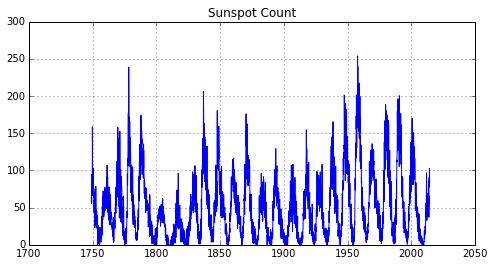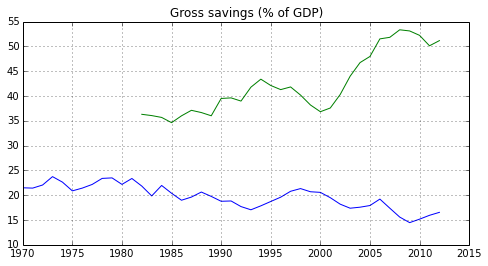# CSV Example¶

Python provides many useful functions to allow us to open data stored in files. For example, the csv module is one useful module for dealing with many forms of data found in the wild. There are many others.

#### Imports¶

In :
import matplotlib.pyplot as plt
import csv

%matplotlib inline

In :
# Let's get a list of available files
%ls -1 *.csv

sunspot_num.csv
WDI_Data_US_China.csv


#### Sunspot Data¶

In :
# Open up the sunspot data and read out the header
sd = open("sunspot_num.csv", "rb")

Out:
['YEAR', 'MON', 'SSN', 'DEV']
In :
# Looks like a simple set of data of monthly counts
# of sunspot activity. Read the rest of the data and
# plot the sunspot data
year = []
spot = []
year.append(float(row["YEAR"]) + (float(row["MON"]) - 1)/12)
spot.append(float(row["SSN"]))

print "Found %d points" % len(year)

Found 3184 points

In :
# Plot the data
plt.figure(figsize=(8,4))
plt.plot(year, spot)
plt.title("Sunspot Count")
plt.grid()#### Savings Rates¶

In :
# Open up the World Bank Data
# I had the full data set for the demo, but for the repository, I only
# included a scrubbed subset of the data I was using. If you'd like to
# use the full data set, you can find it at the link I provide in the
# "Resources" section.
#wd = open("WDI_Data.csv", "rb")
wd = open("WDI_Data_US_China.csv", "rb")

Out:
['Country Name',
'Country Code',
'Indicator Name',
'Indicator Code',
'1960',
'1961',
'1962',
'1963',
'1964',
'1965',
'1966',
'1967',
'1968',
'1969',
'1970',
'1971',
'1972',
'1973',
'1974',
'1975',
'1976',
'1977',
'1978',
'1979',
'1980',
'1981',
'1982',
'1983',
'1984',
'1985',
'1986',
'1987',
'1988',
'1989',
'1990',
'1991',
'1992',
'1993',
'1994',
'1995',
'1996',
'1997',
'1998',
'1999',
'2000',
'2001',
'2002',
'2003',
'2004',
'2005',
'2006',
'2007',
'2008',
'2009',
'2010',
'2011',
'2012',
'2013']
In :
# Looks like rows of data where each row represent an indicator
# for a particular country. And we have data that can range
# from 1960 to 2013. Let's gather all the rows for USA and China.
# Be patient. This requires a little work. We will convert
# each row into a dictionary that can be access by field name.
usa = []
china = []
if row["Country Code"] == "USA":
usa.append({f: row[f] for f in csv_reader.fieldnames})
elif row["Country Code"] == "CHN":
china.append({f: row[f] for f in csv_reader.fieldnames})

In :
# How many indicators are there?
print len(usa), len(china)

1334 1334

In :
# Whoa! That's a lot of data. Let's find all the rows that
# have something to do with savings.
usa_saving = []
for row in usa:
if row["Indicator Name"].lower().find("savings") != -1:
usa_saving.append(row)

china_saving = []
for row in china:
if row["Indicator Name"].lower().find("savings") != -1:
china_saving.append(row)

print len(usa_saving), len(china_saving)

30 30

In :
# That's better. Let's print out the savings indicators.
for s in usa_saving:
print s["Indicator Name"], ", ", s["Indicator Code"]

Adjusted net savings, excluding particulate emission damage (% of GNI) ,  NY.ADJ.SVNX.GN.ZS
Adjusted net savings, excluding particulate emission damage (current US$) , NY.ADJ.SVNX.CD Adjusted net savings, including particulate emission damage (% of GNI) , NY.ADJ.SVNG.GN.ZS Adjusted net savings, including particulate emission damage (current US$) ,  NY.ADJ.SVNG.CD
Adjusted savings: carbon dioxide damage (current US$) , NY.ADJ.DCO2.CD Adjusted savings: consumption of fixed capital (% of GNI) , NY.ADJ.DKAP.GN.ZS Adjusted savings: consumption of fixed capital (current US$) ,  NY.ADJ.DKAP.CD
Adjusted savings: education expenditure (current US$) , NY.ADJ.AEDU.CD Adjusted savings: energy depletion (% of GNI) , NY.ADJ.DNGY.GN.ZS Adjusted savings: energy depletion (current US$) ,  NY.ADJ.DNGY.CD
Adjusted savings: mineral depletion (current US$) , NY.ADJ.DMIN.CD Adjusted savings: natural resources depletion (% of GNI) , NY.ADJ.DRES.GN.ZS Adjusted savings: net forest depletion (% of GNI) , NY.ADJ.DFOR.GN.ZS Adjusted savings: net forest depletion (current US$) ,  NY.ADJ.DFOR.CD
Adjusted savings: net national savings (current US$) , NY.ADJ.NNAT.CD Adjusted savings: particulate emission damage (% of GNI) , NY.ADJ.DPEM.GN.ZS Adjusted savings: particulate emission damage (current US$) ,  NY.ADJ.DPEM.CD
Gross domestic savings (% of GDP) ,  NY.GDS.TOTL.ZS
Gross domestic savings (constant LCU) ,  NY.GDS.TOTL.KN
Gross domestic savings (current LCU) ,  NY.GDS.TOTL.CN
Gross domestic savings (current US$) , NY.GDS.TOTL.CD Gross savings (% of GDP) , NY.GNS.ICTR.ZS Gross savings (% of GNI) , NY.GNS.ICTR.GN.ZS Gross savings (current LCU) , NY.GNS.ICTR.CN Gross savings (current US$) ,  NY.GNS.ICTR.CD

In :
# Let's look at "Gross savings (% of GDP)"
# Using the Indicator Code
usa_year = []
usa_rate = []
for s in usa_saving:
if s["Indicator Code"] == "NY.GNS.ICTR.ZS":
for Y in range(1960, 2014):
# Ignore years without data
if len(s[str(Y)]):
usa_year.append(Y)
usa_rate.append(float(s[str(Y)]))

china_year = []
china_rate = []
for s in china_saving:
if s["Indicator Code"] == "NY.GNS.ICTR.ZS":
for Y in range(1960, 2014):
# Ignore years without data
if len(s[str(Y)]):
china_year.append(Y)
china_rate.append(float(s[str(Y)]))

plt.figure(figsize=(8,4))
plt.title("Gross savings (% of GDP)")
plt.plot(usa_year, usa_rate, china_year, china_rate)
plt.grid()# Resources¶

Data collected from:

 sunspot_num.csv http://solarscience.msfc.nasa.gov/SunspotCycle.shtml WDI_Data_US_China.csv http://data.worldbank.org/data-catalog/world-development-indicators?cid=GPD_WDI